Next: Numerical Solution Up: The Chaotic Pendulum Previous: Basic Problem

Analytic Solution

Before attempting a numerical solution of the equations of motion of any dynamical system, it is a good idea to, first, investigate the equations as thoroughly as possible via standard analytic techniques. Unfortunately, Equations (1237) and (1238) constitute a non-linear dynamical system--because of the presence of the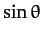term on the right-hand side of Equation (1238). This system, like most non-linear systems, does not possess a simple analytic solution. Fortunately, however, if we restrict our attention to small amplitude oscillations, such that the approximation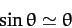(1239)

is valid, then the system becomes linear, and can easily be solved analytically.

The linearized equations of motion of the pendulum take the form: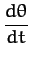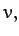(1240)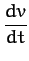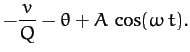(1241)

Suppose that the pendulum's position,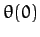, and velocity,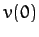, are specified at time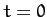. As is well-known, in this case, the above equations of motion can be solved analytically to give: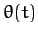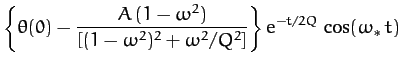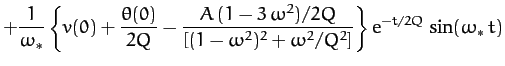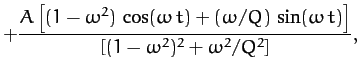(1242)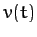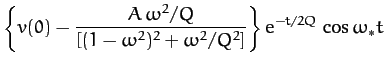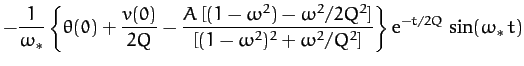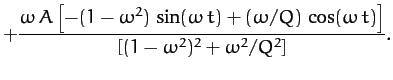(1243)

Here,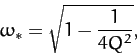(1244)

and it is assumed that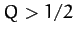. It can be seen that the above expressions forand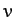both consist of three terms. The first two terms clearly represent transients, since they depend on the initial conditions, and decay exponentially in time--see Section 3.9. In fact, the e-folding time for the decay of these terms is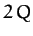(in normalized time units). The final term represents the time-asymptotic motion of the pendulum, and is manifestly independent of the initial conditions--see Section 3.5.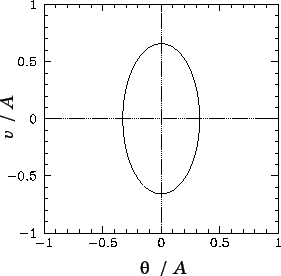It is often convenient to visualize the motion of a dynamical system as an orbit, or trajectory, in phase-space, which is defined as the space of all of the dynamical variables required to specify the instantaneous state of the system. For the case in hand, there are two dynamical variables,and, and so phase-space corresponds to the-plane. Note that each different point in this plane corresponds to a unique instantaneous state of the pendulum. [Strictly speaking, we should also consider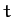to be a dynamical variable, since it appears explicitly on the right-hand side of Equation (1238).]

It is clear, from Equations (1242) and (1243), that if we wait long enough for all of the transients to decay away then the motion of the pendulum settles down to the following simple orbit in phase-space: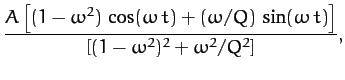(1245)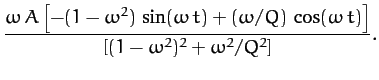(1246)

This orbit traces out the closed curve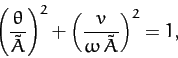(1247)

in phase-space, where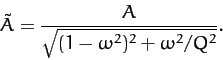(1248)

As illustrated in Figure 58, this curve is an ellipse whose principal axes are aligned with theandcoordinate axes. Observe that the curve is closed, which suggests that the associated motion is periodic in time. In fact, the motion repeats itself exactly every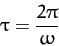(1249)

normalized time units. The maximum angular displacement of the pendulum from its undriven rest position (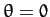) is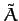. As illustrated in Figure 59, the variation ofwith driving frequency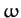[see Equation (1248)] displays all of the features of a classic resonance curve. The maximum amplitude of the driven oscillation is proportional to the quality-factor,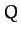, and is achieved when the driving frequency matches the natural frequency of the pendulum (i.e., when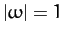). Moreover, the width of the resonance in-space is proportional to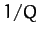--see Section 3.7.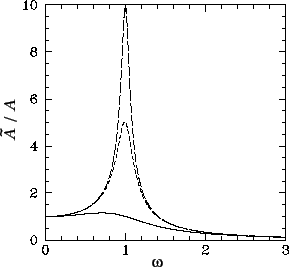The phase-space curve shown in Figure 58 is called a periodic attractor. It is termed an attractor'' because, irrespective of the initial conditions, the trajectory of the system in phase-space tends asymptotically to--in other words, is attracted to--this curve as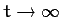. This gravitation of phase-space trajectories towards the attractor is illustrated in Figures 60 and 61. Of course, the attractor is termed periodic'' because it corresponds to motion which is periodic in time.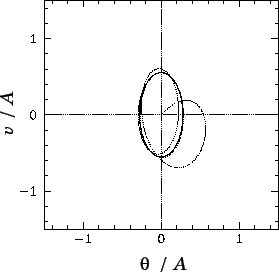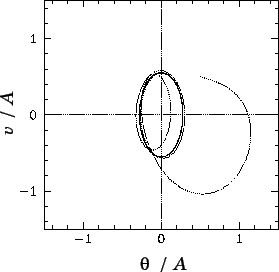Let us summarize our findings so far. We have discovered that if a damped pendulum is subject to a low amplitude periodic drive then its time-asymptotic response (i.e., its response after any transients have died away) is periodic, with the same period as the driving torque. Moreover, the response exhibits resonant behaviour as the driving frequency approaches the natural frequency of oscillation of the pendulum. The amplitude of the resonant response, as well as the width of the resonant window, is governed by the amount of damping in the system. After a little reflection, we can easily appreciate that all of these results are a direct consequence of the linearity of the pendulum's equations of motion in the low amplitude limit. In fact, it is easily demonstrated that the time-asymptotic response of any intrinsically stable linear system (with a discrete spectrum of normal modes) to a periodic drive is periodic, with the same period as the drive. Moreover, if the driving frequency approaches one of the natural frequencies of oscillation of the system then the response exhibits resonant behaviour. But, is this the only allowable time-asymptotic response of a dynamical system to a periodic drive? It turns out that it is not. Indeed, the response of a non-linear system to a periodic drive is generally much richer and far more diverse than simple periodic motion. Since the majority of naturally occurring dynamical systems are non-linear, it is clearly important that we gain a basic understanding of this phenomenon. Unfortunately, we cannot achieve this goal via a standard analytic approach, since non-linear equations of motion generally do not possess simple analytic solutions. Instead, we must employ numerical methods. As an example, let us investigate the dynamics of a damped pendulum, subject to a periodic drive, with no restrictions on the amplitude of the pendulum's motion.Next: Numerical Solution Up: The Chaotic Pendulum Previous: Basic Problem
Richard Fitzpatrick 2011-03-31